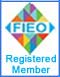Note: No part of this website may be reproduced, stored in a retrieval, or transmitted in any form or by any mean

## Our ProductsHome » Physics Laboratory Instruments   »   Fiber Optic Apparatus Fiber Optic Apparatus Objective To MEASURE Numerical Aperture and Acceptance angle of Optical Fiber. To MEASURE attenuation in Optical Fiber.Fiber Optic Apparatus Code: FOA-001 Fiber Optic ApparatusNumerical aperture is a basic descriptive characteristic of a specific fiber. It is represents the size or degree of openness of the input acceptance cone. Mathematically it is defined as the sine half angle of the acceptance cone. Using Snells law, the maximum angle within which light will be accepted into and guided through fiber iswhereis the numerical aperture and n1 and n2 are the refractive indices of the core and the cladding. In fiber, ideally a ray launched at angleat the input end should come out at the same anglefrom output end. Therefore, the far field at the output end will also appear as a cone of semi angleemanating from the fiber end. The objective of Fiber optic setup is to measure the numerical aperture (NA) of optical fiber. With present setup, NA is measured in two ways. In first procedure the dia (D) of circle formed on the screen placed at some distance (L) from the fiber is measured (as shown below) and NA is calculated using the formulaExperimental Setup 1Experimental Setup 2 In second procedure, screen is replaced by the photo detector and intensity is measured and plotted at different angles (as shown above). Using the intensity plot, NA is calculated. To measure attenauation in optical fiber, output (voltage) is measured at the output of 1 meter optical fiber Then output is measured at the output of 5 meter optical fiber. Attenuation can be calculated using formulaWhere α is loss in nepers/m, L1= Length of shorter cable (1m), L2= Length of longer cable (5m) Instrument The Apparatus consists of Laser Diode, Objective (10X), Fiber (1 mtr long & 5 mtr long), Dectector, Multimeter, Chucks and bases to hold the assemblies.